## Kinetic Theory of GasesEven cold, wintry air has kinetic energy, even if it doesn't feel like it.

(Source)

We use the kinetic theory of gases to peer through the galaxy of the ideal gas law to look at the stars within. Think of it as what the ideal gas law would look like when viewed through a microscope. Instead of considering gases on a macroscopic scale (y'know, people sized), it treats gases as a collection of millions of molecules.

These are the laws that tie everything together: they relate the temperature, pressure, and volume of a gas back to what the individual molecules in the gas are doing. This is good because, the molecules' parents are always asking us what they were doing between 3 and 5 on a weekday afternoon, and we're tired of being put on the spot.

We said before that the temperature of a substance is a measure of how fast its molecules are moving—or in other words, a measure of the average kinetic energy of the molecules. Well, the kinetic theory of gases lets us relate the kinetic energy of the molecules in a gas to the temperature, volume, and pressure of the gas. Which gas? Any gas. Yes, even that one, you stinker.

The kinetic theory of gases makes several assumptions:

1. The gas is made up of a very large number of molecules, N.
2. These molecules are all the same, with the same mass, m, and are moving randomly in all possible directions. Think Powerball.
3. Although there are millions of molecules, they are far apart from each other in relation to their size.
4. We assume that these molecules only interact with each other and the walls of the container, scattering around in many zillions of tiny, adorable collisions.
5. Molecules will collide with each other and with the walls of the container in a perfectly elastic, energy-preserving fashion.

What that last one means is that no energy is lost in the form of heat when the two objects collide. Therefore, after each collision the molecules still have the same kinetic energy. This is important, because if collisions caused molecules to lose kinetic energy, after thousands and billions of collisions, the molecules would have no kinetic energy (speed) at all. This would cause gases to cool into liquids which in turn would cool and solidify over time just because the molecules in them are losing energy in their collisions.

Eventually, the universe would consist of one solitary solid mass at absolute zero. Hope you like your neighbors, because you're stuck with them in the frozen universe ball for the rest of eternity. At least, that's what would happen, if we weren't making that last assumption.

With these five assumptions in mind, let's start thinking about how much force one gas molecule exerts on the wall of the box as it collides. This involves a lot of math, for better or worse. We can memorize the final equations, but taking the time to understand where these equations come from not only helps us remember them, but also helps us understand the physics they describe. Sounds like a good deal to us.

### March of the Kinetic Theory of Gases

So brace for impact. Here comes the march of the equations,(and we promise it's nothing like the march those poor emperor penguins endure, and it won't take 20 days and 20 nights either).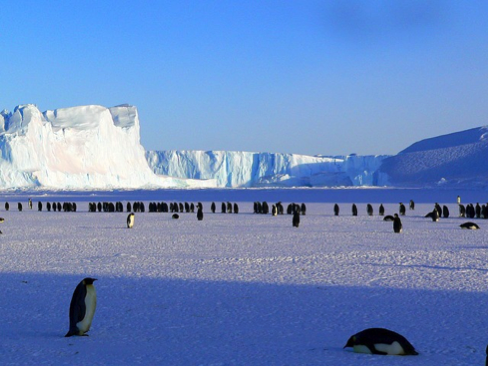"Are we there yet?"

(Source)

Remember Newton's second law? It's the one that says that the rate of change of the momentum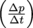of a particle or body is equal to the net force it experiences (F). Equated out, it appears as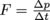.

It may not look like much, but it's why little kids would much rather have Hulk Hogan give them a push from the top of the sledding hill, rather than have us do it. Junior's sled will go a lot faster, spiking a much larger change in momentum over the same amount of time, because Mr. Hogan can (and will) exert a much larger force on the sled than we could ourselves.

This all ties into the momentum of an object. We learned in the Momentum Chapter that linear momentum, px, depends on the mass of an object, m, and its speed in one dimension, vx. The equation is px = mvx, While molecular momentum is admittedly negligible compared to speeds we're used to dealing with, to each other the molecules have some collisions to fear. Would you rather be, say, a relatively large and heavy carbon dioxide molecule or helium (the lightweight)?

Let's think about it on a macroscopic level for comparison. What would you rather get hit by? A fly buzzing 15 miles per hour or a car driving 15 miles per hour? We pick the fly, because even though it is going the same speed as the car, it's just a bit smaller, so it has much less momentum and will do a lot less damage when it smashes into us.

The change in momentum for a molecule is nothing but Δpx = m1vx1 – m2vx2 just as it is for the macroscopic objects we used in the momentum chapter We're going to assume that the molecule hits the wall and bounces directly backward, sending the molecule back along the same path that it was traveling when it hit the wall, but in the opposite direction. So m1v1 = -m2v2. Now our equation looks like this: Δpx = m1vx1 – (-m2vx2).

Our assumption number 5 said that the molecules collide in an elastic fashion with no energy or momentum lost. This means that the mass of the object stays the same, so m1 = m2, and the velocity stays the same as well, so vx1 = vx2. The more things change, the more they stay the same, eh?

This allows us to rewrite the formula yet again as Δpx = m1vx1 – (-m1vx1) = 2m1vx1 = 2mvx. Just to make sure we're all on the same page, this is the change in momentum that one molecule experiences when it hits the wall of the container once.

But the air molecules in our balloon example are constantly colliding with the walls of the container. After a molecule hits one side of a balloon with diameter d, it takes it a time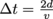before it hits that wall again. So if a balloon is 10 cm wide and an air molecule is moving at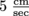, then it will take the air molecule 2 seconds to make a round trip. If the balloon is 100 cm wide, then it will take the molecule 20 seconds to complete the entire trip. Never fear: molecules are a lot faster than snails in reality.

Let's plug these new values, Δp = 2m vx and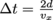, into our force equation,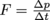. After substitution, it looks like this: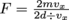. Fortunately for all the equation memorizers out there, this mess simplifies to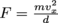for a single molecule.

### Don't Celebrate Yet

Hold up, we're forgetting something. Our balloon contains N molecules of gas, not just one molecule. Assumption (2) says that they are all identical in mass, so the force the balloon experiences due to N molecules, each of mass, m, moving at average velocity vavg, is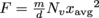, where N is the total number of molecules in the system. We're going to look at just the x-direction for the change in momentum.No! No more balloons!

(Source)

Phew. All of the different parts of physics we've learned thus far—momentum, force, energy—come together to explain a completely new phenomenon; how the temperature, pressure, and volume of a gas relate to the movement of molecules in a gas. Who's good? We're good. Aw yeah.Like the header says, though, don't celebrate yet. We still have to relate the energy of the gas molecules to the temperature, pressure, and volume of the gas. All we've done so far is figure out how much force they exert on the balloon. We'll get to the energy by way of pressure.When something is under pressure, we're talking about how much force is exerted over a given (or measurable) area, or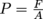. Innocuous, as far as equations go, but what does it mean?

Let's do an experiment. Take your right index finger and gently poke the palm of your left hand. Now push down as hard as you can. After that, take the palm of your right hand and push down on your left hand as hard as you can. Even though you're pushing as hard as you can in both examples, and so exerting the same force, pushing with one finger is a lot more uncomfortable. That's because the area over which we exert pressure matters. And now we need an ice pack.Before we can plug our force equation,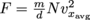, into the pressure equation, we have to take care of one more tiny thing. This equation is describing force in one dimension, x. But the molecules in our balloon are moving in all three dimensions. Since the molecules are moving randomly, they are just as likely to move in the x, y, or z directions and their average speed should be the same in all directions, so we'll say that vxavg2 = vyavg2 = vzavg2.

The total square velocity is the sum of all three directions added together, or vavg2 = 3vxavg2.The force equation,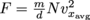, then becomes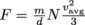. Plugging this into the pressure equation,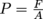, we get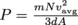, which becomes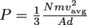.

Voilà! This is how the motion of lots of tiny air molecules jiggling about in a balloon relates to the pressure in the balloon. This is actually pretty amazing. We can relate the speed of these teeny tiny particles we can't even see to the pressure they exert, something we experience every day. Can you feel your mind expanding (or is it just getting hotter)?

### Keep Up the Pressure

Great. So we got the pressure, but our goal from the start was to find a way to relate this stuff to energy. Are we any nearer to finding the energy of the molecules in the gas? Are we almost there yet? We think so.We can simplify our equation some by relating it to the volume, V, of the container it's in. Since the volume of a square container is nothing more than area, A, times length, d, we next have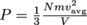.

This next step is going to seem strange at first, but there's a madness to our madness. Er, we mean a method to our method. Wait. Whatever, the equation can now be rearranged into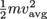.

That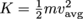part we snuck in there should look familiar. This is the average kinetic energy, Kavg. Since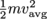, we can solve for the kinetic energy of the gas in terms of its pressure and volume: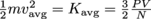.

We're in the home stretch here. From the ideal gas law, we know that PV = NkT, so we have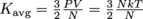.

N cancels out to leave us with the final equation, the one we've been looking for. We'll just stick it on its own line, make it really stand out from the crowd.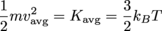We did it! We found the energy for molecules in a gas. Can we get a celebratory booyah?

The above equation is for the average kinetic energy of a single molecule in the gas. There's more to energy than kinetic energy, though, as we all well know. The total internal energy of some amount of an ideal gas, U, relates to the average kinetic energy of each molecule, Kavg, and the total number of particles, N, in the gas as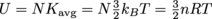.

All of that derivation gave us two important equations that tell us how individual molecules randomly flopping about relate to the temperature and volume of the gas that they make up.

Volume: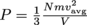Temperature: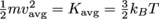Lastly, we rearrange this last equation to solve for the average speed of molecules in the gas, called the root-mean-square (rms) velocity, vrms.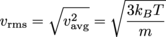This last equation (yes, it's true!) can also be written relative to the molar mass, M, or the mass of one mole of a gas,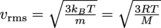.

Now we can calculate just how fast the Helium atoms are zipping around in a balloon whether they're at room temperature, it's a hot summer day, or they're chilling with the penguins at the North Pole.

This section contains a lot of equations to help us relate the pressure, temperature, and volume of ideal gases to the speed, vavg, and the average kinetic energy, Kavg, of the gas molecules. The important equations for physics midterm questions are below. First off, we've got equations for pressure.These two equations are essentially the same statement. The conversion from one to the other uses volume, V = Ad, so we only have to memorize one of these equations as long as we remember that volume is area times distance.

Next on the summary docket, kinetic energy.Squint at these two equations for a bit, and you'll that they are twinsies, too. You'll also be kind of rude; it's not polite to stare at people, y'know? If we rewrite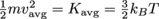as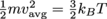and solve for vavg,, then we get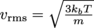.

Again, don't go memorizing both equations, unless you've got a bunch of spare brain cells lying around with nothing to do.

Finally, the interval energy of a gas offers us two equivalent forms.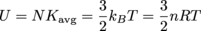And that's a wrap. As usual, when solving a problem, always choose the form of an equation best fits the problem, rather than banging your head against a wall trying to get another equation to do the job. The wall won't appreciate the extra kinetic energy, and neither will you.

### Common Mistakes

Be careful when converting temperatures, volumes, and masses to the correct units. If the equation involves a constant, such as the Boltzman constant, the units of the constant reveal something about what the units for the rest of the variables should be. The Boltzman constant's units are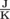. That means temperatures should be rockin' it Kelvin-style, not in Celsius.